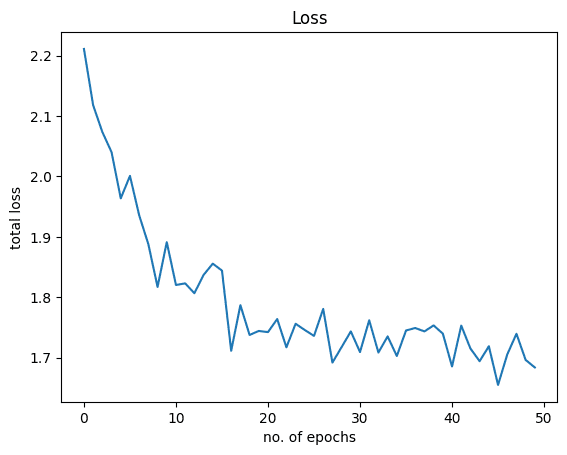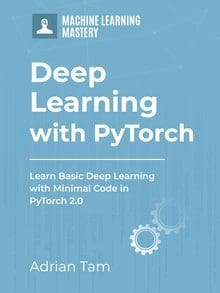# Building a Logistic Regression Classifier in PyTorch

Last Updated on April 8, 2023

Logistic regression is a type of regression that predicts the probability of an event. It is used for classification problems and has many applications in the fields of machine learning, artificial intelligence, and data mining.

The formula of logistic regression is to apply a sigmoid function to the output of a linear function. This article discusses how you can build a logistic regression classifier. While previously you have been working on a single-varable dataset, here we’ll be using a popular MNIST dataset to train and test our model. After going through this article, you’ll learn:

• How to use logistic regression in PyTorch and how it can be applied to real-world problems.
• How to load and analyze torchvision datasets.
• How to build and train a logistic regression classifier on image datasets.

Kick-start your project with my book Deep Learning with PyTorch. It provides self-study tutorials with working code.

Let’s get started.Building a Logistic Regression Classifier in PyTorch.
Picture by Catgirlmutant. Some rights reserved.

## Overview

This tutorial is in four parts; they are

• The MNIST Dataset
• Build the Model with nn.Module
• Training the Classifier

## The MNIST Dataset

You will train and test a logistic regression model with MNIST dataset. This dataset contains 6000 images for training and 10000 images for testing the out-of-sample performance.

The MNIST dataset is so popular that it is part of PyTorch. Here is how you can load the training and testing samples of the MNIST dataset in PyTorch.

The dataset will be downloaded and extracted to the directory as below.

Let’s verify number of training and testing samples in the dataset.

It prints

Each sample in the dataset is a pair of image and label. To inspect the data type and size of the first element in the training data, you can use type() and size() methods.

This prints

You can access samples from a dataset using list indexing. The first sample in the dataset is a FloatTensor and it is a $28\times 28$-pixel image in grayscale (i.e., one channel), hence the size [1, 28, 28].

Now, let’s check the labels of the first two samples in the training set.

This shows

From the above, you can see that the first two images in the training set represent “5” and “0”. Let’s show these two images to confirm.

You should see these two digits:Usually, you do not use the dataset directly in training but through a DataLoader class. This allows you to read data in batches, not samples.

In the following, data is loaded into a DataLoader with batch size at 32.

### Want to Get Started With Building Transformer Models with Attention?

Take my free 12-day email crash course now (with sample code).

Click to sign-up and also get a free PDF Ebook version of the course.

## Build the Model with nn.Module

Let’s build the model class with nn.Module for our logistic regression model. This class is similar to that in the previous posts but the numbers of input and output are configurable.

This model will take a $28\times 28$-pixel image of handwritten digits as input and classify them into one of the 10 output classes of digits 0 to 9. So, here is how you can instantiate the model.

## Training the Classifier

You will train this model with stochastic gradient descent as the optimizer with learning rate 0.001 and cross-entropy as the loss metric.

Then, the model is trained for 50 epochs. Note that you have use view() method to flatten the image matrices into rows to fit the same of the logistic regression model input.

During training, you should see the progress like the following:

You have achieved an accuracy of around 86% by training the model for only 50 epochs. Accuracy can be improved further if the model is trained longer.

Let’s visualize how the graphs for loss and accuracy look like. The following is the loss:And this is for accuracy:Putting everything together, the following is the complete code:

## Summary

In this tutorial, you learned how to build a multi-class logistic regression classifier in PyTorch. Particularly, you learned.

• How to use logistic regression in PyTorch and how it can be applied to real-world problems.
• How to load and analyze torchvision datasets.
• How to build and train a logistic regression classifier on image datasets.

## Get Started on Deep Learning with PyTorch!#### Learn how to build deep learning models

...using the newly released PyTorch 2.0 library

Discover how in my new Ebook:
Deep Learning with PyTorch

It provides self-study tutorials with hundreds of working code to turn you from a novice to expert. It equips you with
tensor operation, training, evaluation, hyperparameter optimization, and much more...

### 7 Responses to Building a Logistic Regression Classifier in PyTorch

1.Eduardo Passeto January 4, 2023 at 10:45 pm #

Thank you for the excellent tutorial!

•James Carmichael January 5, 2023 at 7:17 am #

You are very welcome Eduardo! We appreciate your feedback and support.

2.Alberto Gil March 14, 2023 at 1:25 am #

Nice and very didactic tutorial, thanks!

But I have a question. Torch CrossEntropyLoss is used, that includes internally a softmax step. So, this is rather an example of softmax classification (appropriate to multi class) than logistic regression one (appropriate to binary classification).

In fact I get better accuracy if I remove the sigmoid step at the forward function.

As I am a newcomer in this field, can you comment on this?

•James Carmichael March 14, 2023 at 9:07 am #

Hi Alberto…You are correct! Binary classification is a subset to multiclass classification so your results make sense.

3.Marco F May 6, 2023 at 11:20 am #

I think that this implementation is wrong.
The sigmoids should be removed and CrossEntropyLoss should be replaced with BCEWithLogitsLoss.
In fact, CrossEntropyLoss internally applies the softmax while BCEWithLogitsLoss internally applies sigmoid.

•James Carmichael May 7, 2023 at 5:40 am #

Hi Marco…Thank you for your feedback! Can you provide more detail as to the results you are receiving that confirm that the implementation is wrong?

•Marco F May 9, 2023 at 2:14 pm #

Hi James,

If you look at the CrossEntropyLoss pydoc (https://pytorch.org/docs/stable/generated/torch.nn.CrossEntropyLoss.html) you see that the input is expected to be the unnormalized logits for each class. So, basically, in a simple single dense layer network, it should be the output of a linear layer. In your example, instead, the input is the output of a sigmoid. This last step is not needed since it is already applied by CrossEntropyLoss. Your example is pratically like y=loss(softmax(sigmoid(x)).